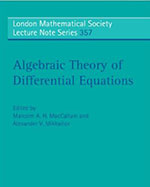Algebraic Theory of Differential Equations (London Mathematical Society Lecture Note Series) - Maplesoft Books - Maple Books, Maple Resources and Math BooksHome : Maplesoft Books : Algebraic Theory of Differential Equations (London Mathematical Society Lecture Note Series)Details Search# Algebraic Theory of Differential Equations (London Mathematical Society Lecture Note Series)

## 2009